## … and why math (alone) won’t help us

I was very happy to speak at Curry On once again. Last year, following our work with Quasar, I spoke on continuations vs. monads. This year I gave a talk about computational complexity results in software verification, a subject I became interested in while formally specifying and verifying our upcoming revamped version of Galaxy.

What follows is an extended version of the talk (embedded above), containing some additional proofs and results I didn’t have time to cover, complete with footnotes, for those wishing to study the topics further. Reading time: ~45 mins.

In this article, we try to understand the relationship between programs and correctness, and in particular, why writing correct programs is hard. We will review results from computability theory and complexity theory, and see that programs and complete understanding – which is required for correctness – are fundamentally at odds. The main contribution of this text is aggregating in one place some results (as well as some references) pertaining to the essential difficulty of producing correct software. My motivation was various statements I found online (such as this, although it is far from the only one) claiming that software correctness is easy to achieve with the right tools. While some tools may (and do) certainly assist ensuring programs are correct, correctness comes at a significant cost. The cost of correctness – like the energy cost of reducing entropy – is a result of the “natural laws” of computation, that cannot possibly be avoided.

## CHAPTER I: FOUNDATIONS

The story of theoretical CS began about a hundred years ago. With the growing success of mathematical logic, the mathematician David Hilbert laid out a program to formalize all of mathematics. He wanted to prove the formalism consistent and complete, and to find a decision procedure, an algorithm for determining the truth of every formal statement:

• Consistency: proof that no contradiction can be obtained in the formalism.
• Completeness: all true mathematical statements can be proved in the formalism.
• Decidability: an algorithm deciding whether any statement is a theorem (Entscheidungsproblem)

Hilbert believed that anything that can be formally reasoned can also be fully understood, and by that I mean all of its properties proven. the 19th century view on the limits of knowledge was expressed by the maxim “ignoramus et ignorabimus”, meaning “we do not know and we will not know”. For his retirement in 1930, Hilbert gave a lecture – “Naturerkennen und Logik” (Logic and the understanding of nature) – in Königsberg to the Society of German Scientists and Physicians which he challenged this view. He said:

For the mathematician there is no Ignorabimus, and, in my opinion, not at all for natural science either. … The true reason why [no one] has succeeded in finding an unsolvable problem is, in my opinion, that there is no unsolvable problem. In contrast to the foolish Ignorabimus, our credo avers: We must know, we shall know!1

Those words – Wir müssen wissen. Wir werden wissen – are inscribed on his tombstone.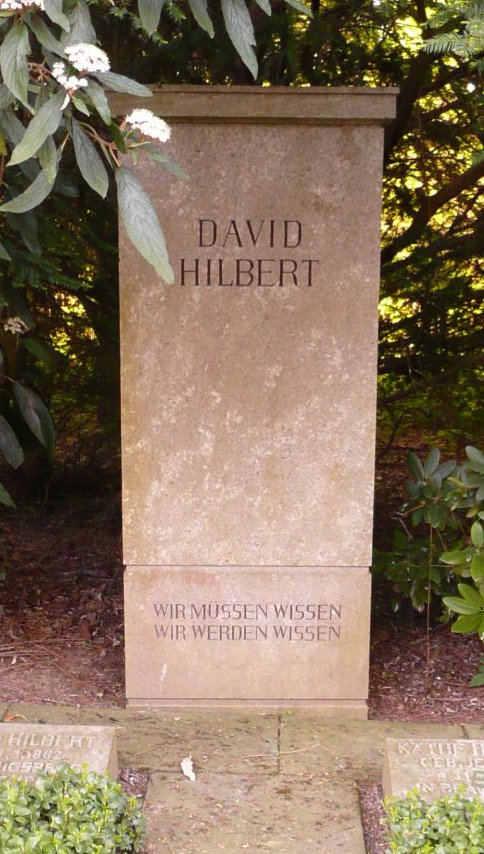Unbeknownst to Hilbert at the time, his program had been demolished a day earlier, when Kurt Gödel tentatively announced his first incompleteness result2.

Gödel’s incompleteness theorems demolished the first two pillars of Hilbert’s program (some say also the third, only he didn’t realize it3), and in 1936, Church and Turing broke the third, that of the decision problem, with proofs of what is today known as the halting theorem.

The halting theorem – CS’s first theorem – was proven in both Alonzo Church’s4 and Alan Turing’s5 papers in 1936. The theorem is so fundamental to the essence of computation that it is no accident that it appeared alongside both formulations of computation. It states that there cannot exist an algorithm that decides whether any given program ever terminates. The proof (which is given here in a shortened form) is based on the idea of diagonalization, or self reference, the same idea Gödel had used in his incompleteness proof.

Assume such a program H exists:

H(M, x) := IF M halts on x THEN TRUE
ELSE FALSE


we can then easily construct $G$:

G(y) := IF H(y, y) = FALSE THEN TRUE
ELSE loop forever


If we then pass $G$ to itself ($G(G)$) we get a contradiction between what $G$ does and what $H$ says that $G$ does.

It is interesting to note that the halting theorem immediately yields a version of Gödel’s first incompleteness theorem. The proof says simply6: suppose that for every true statement there was a logical proof in some logical theory (basically a set of axioms). So, given a program, starting from those axioms, we can enumerate all theorems one by one by using logical deduction. Because the number of all statements, let alone all theorems, is countable, and because every statement either has a proof or its negation does, we would eventually find the proof of one or the other. Therefore, given a program, we can start enumerating all theorems until we find a theorem showing that the program terminates, or one that says it doesn’t, thus violating the halting theorem.

However, the halting theorem has some other immediate corollaries that tie it more directly to the problem of software verification, which is our main focus. The first is the simple result about reachability.

There does not exist a program R, when given a program M, a set of initial states I and state configuration S, determines whether or not M ever reaches S when it starts in one of the states in I.

The proof is a simple reduction from halting: if there were an such an algorithm, we could ask it if, starting with the input x it ever reaches the halting state, and so solve the halting problem7.

A more interesting corollary is Rice’s Theorem. Programs can compute what’s known as partial functions. If they stop when given an input, we consider the output written on the tape to be mapped to the initial input; if they never stop for a given input, we consider that input unmapped. Rice’s theorem states this:

For any non-trivial property of partial functions, no algorithm can decide whether or not a program (TM) M computes a partial function with that property.

For example, there is no algorithm to determine whether the output of a program is always the square of its input. This theorem is also proven by a reduction from Halting.

For our purposes, I think that the most interesting corollary of the halting theorem is what we’ll call the “Busy Beaver theorem”8, because it captures best the notion of the complexity of analyzing programs, giving it some quantitative measure. Like all results we have and will cover here, this one applies to both dynamic and static views of programs (namely, their behavior as they run or their global properties), but this one targets each view separately, and so it helps give a more direct intuition that they are two sides of the same coin.

In the dynamic version, we define the following function on the natural numbers:

$f(n)$ = the maximum number of steps a halting TM with $n$ control states may take before halting

A Turing machine’s control states constitute its program, so $n$ is the size of the program. This function is well defined because there is a finite number of TMs of size $n$. The subset of them that terminates is also finite, so there exists a maximal number of states.

The theorem says $f$ is non-computable. Suppose that $f$ were computable. If so, that would be a violation of the halting theorem. Given a TM, we count the number of control states, $n$, compute $f(n)$ (which is possible by our assumption of $f$’s computability), and then run the machine for $f(n)$ steps. If it doesn’t terminate by then — then it must surely never terminate (by the definition of $f$), and we have a decidable procedure of the halting problem, in contradiction with the halting theorem.

Not only is $f$ a non-computable function, but any function that is known to be greater than $f$ for all $n$ is also non computable, because if we knew of such a function, the same procedure would again yield a decision for halting.

The very same argument applies to a static view of programs. To understand this result, we need to know that given a logical theory with a finite set of axioms, an algorithm can apply deduction rules one by one starting with the axioms, and start enumerating all theorems (provable statements) one by one.

This time, we’ll define the function

$g(n)$ = maximum size of a minimal proof of termination of a halting TM of size $n$

For every terminating TM of size $n$, we pick the shortest proof of termination (there must be at least one proof, as a terminating trace is such a proof). We then take the maximum among all terminating TMs of size $n$ (of which there’s a finite number).

Similarly to $f$, $g$ must also be non-computable. If $g(n)$ were computable, then given a TM of size $n$, we’d describe the machine with some axioms and then we would enumerate all theorems with proofs of size $\leq g(n)$ — that’s a finite number — and if no proof is found, we’d know the machine to never terminate; again, in contradiction with the halting theorem.

This corollary of the halting theorem captures the following fact: the function tying the size of a program to the difficulty of proving that it terminates, regardless of whether we’re interested in a dynamic proof technique or a static one, is greater than all computable functions (more precisely: there is no computable function that can be known to be greater than our function for all values of $n$).

While we cannot compute $f$ for all $n$, we can compute it, or lower bounds for it, for some values. For $n = 6$ (and a 2-symbol alphabet), it is $> 7.4 \times 10^{36534}$.

Another question we may ask is this: we know that we can’t come up with an algorithm to decide whether any arbitrary program halts, but are there specific programs that we cannot know whether they halt or not (even if we tailor a decision procedure specifically for them)?

Gödel’s second incompleteness theorem yields such a program. The theorem states it is impossible for a logical system to prove its own completeness. The logical system that is widely accepted as the foundation of mathematics is called ZFC — Zermello-Fraenkel set theory with the axiom of choice; within that system we can prove most mathematical theorems we care about (with some very notable, very special exceptions). So we can construct a program that does the following: starting from the ZFC axioms, enumerate all theorems and stop when you reach a contradiction (e.g. 1 = 2). The program would stop if-and-only-if ZFC is inconsistent. We assume ZFC to be consistent, and so would expect the program to never halt, but we can’t prove it, by the second incompleteness theorem.

This past May, Adam Yedidiya and Scott Aaronson published a program that compiles down to a Turing machine that works by that principle (or something equivalent to it)9. The result was quickly improved upon by Stefan O’Rear, who created a Turing machine with 1919 control states which cannot be proven to terminate (i.e., it is “independent of ZFC”). This machine is so small that it comfortably fits in a 4K challenge; it is comparable to a Python program with 100-200 lines.

This mean that there are small programs — just a couple hundred lines or less — whose behavior cannot be mathematically determined. Not because the analysis would be too large or intractable, but because mathematics is not powerful enough to ever prove the behavior of this program, even in theory.

## Computer Science is not Math

In any event, with the work of Gödel, Church and Turing, Hilbert’s program — at least as originally planned — was laid to rest, 80 years ago by simple, compact, mathematical objects that can be formally reasoned about, but not fully understood: computer programs.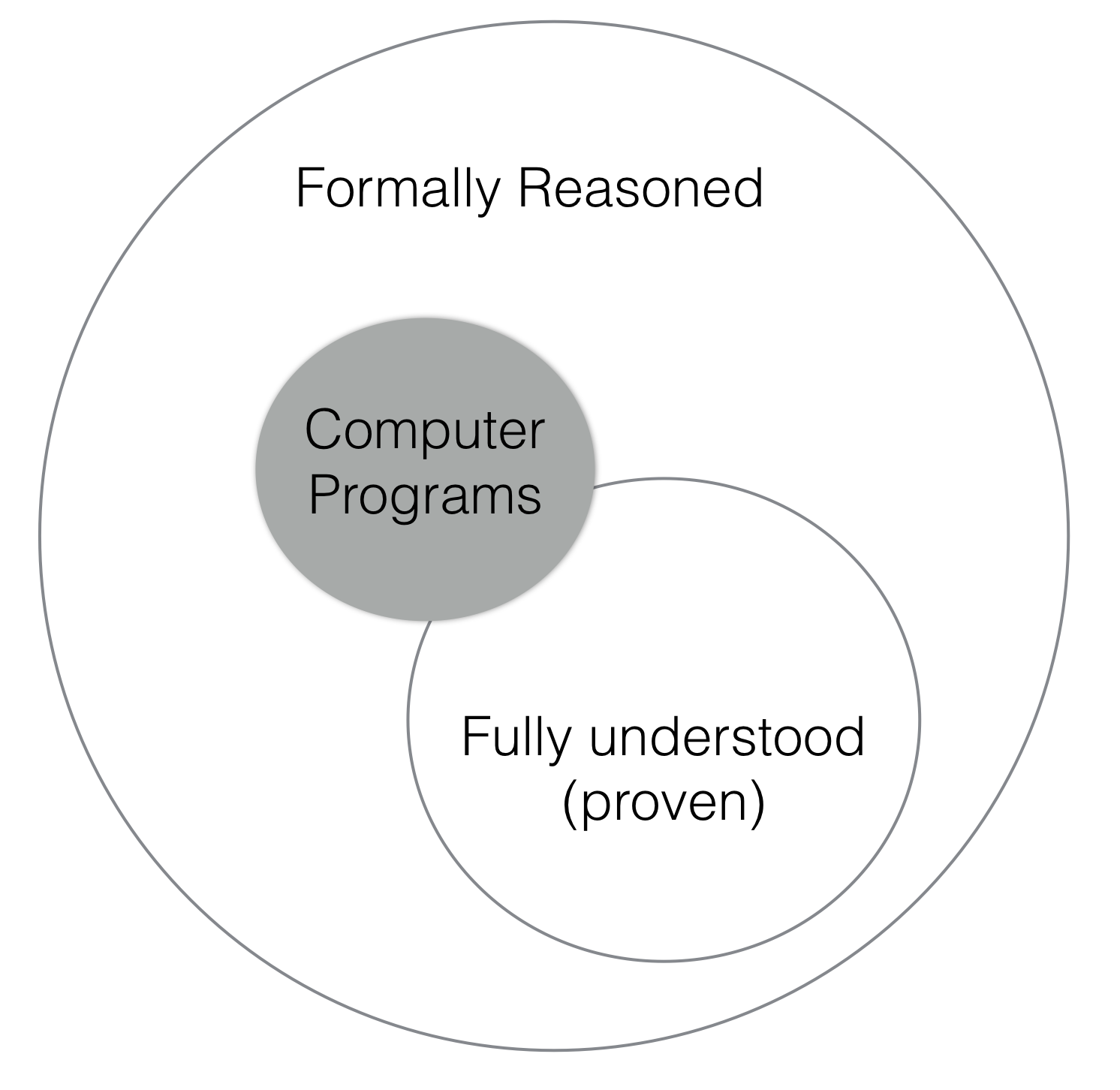There is one foundational question to be asked. The entire premise of the halting theorem rests on the idea that a function that requires infinite computational steps is uncomputable. This isn’t entirely obvious. After all, math often handles infinite objects10. While Church glossed over this point (perhaps believing it to be obvious — he called it a “vague intuitive notion”) Turing, whose paper was much more philosophical than Church’s, made a point of explaining that an algorithm is something that is carried out by a person or by a machine.

In 1947, he gave a lecture to the London Mathematical Society. “Some years ago”, he said,

I was researching on what might now be described as an investigation of the theoretical possibilities and limitations of digital computing machines.

It was essential in these theoretical arguments that the memory should be infinite… Machines such as the ACE may be regarded as practical versions of this same type of machine11.

This is how Turing viewed his 1936 breakthrough achievement. While the mathematical formalization may appeal to the infinite, it was clear to Turing that the notion of computation is tied to some physical process. If that were not so, there would be no justification for the centrality of the halting problem and its use as a negative solution to Hilbert’s Entscheidungsproblem. An algorithm is something that is carried out in the physical world.

The Church-Turing thesis, as it is presented today, conjectures that any computation done by any realizable physical process can be computed by the universal computational models described by Church and Turing. In a sense, this means that everything in the universe could be formalized and simulated as a computer program.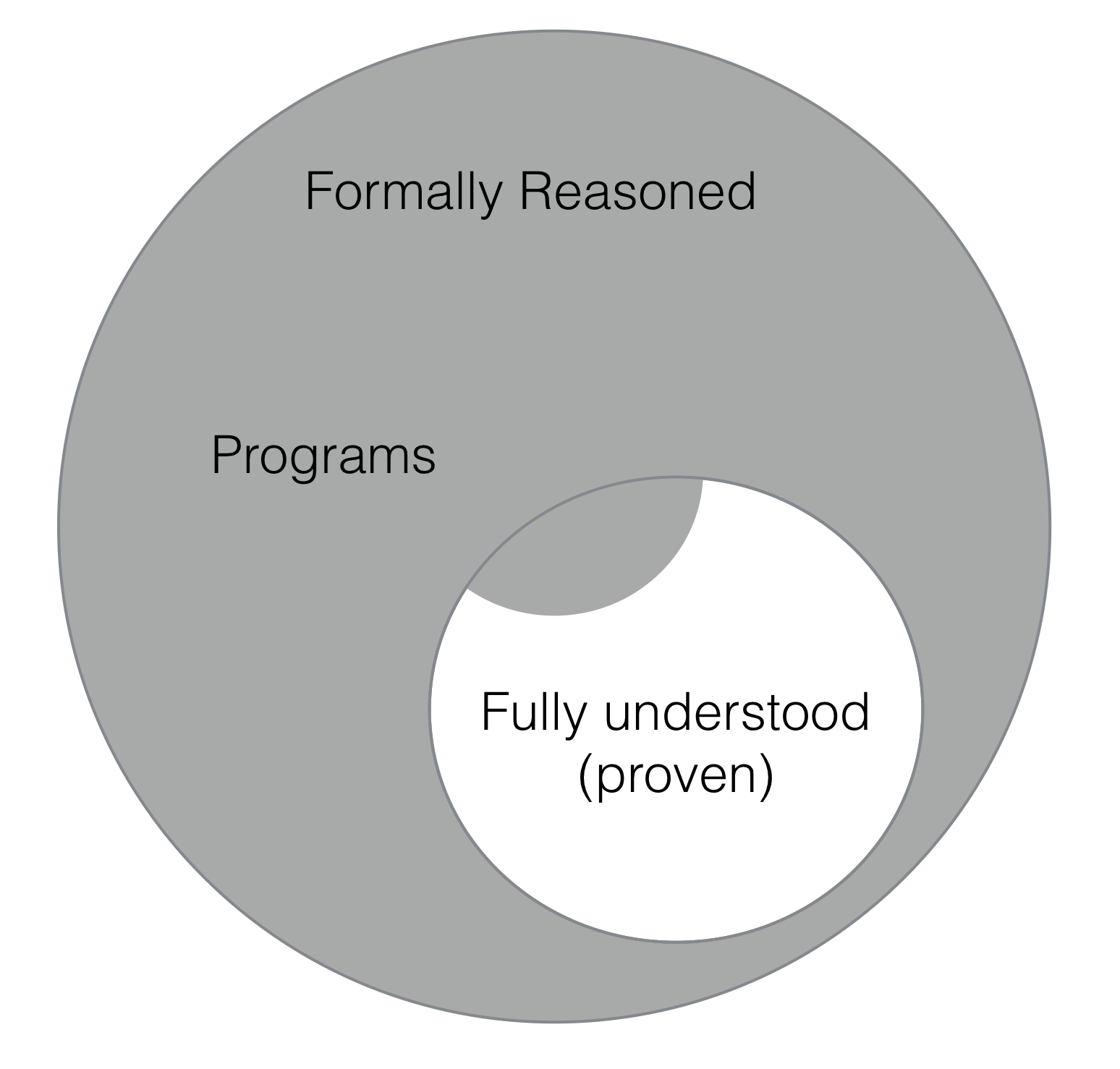Programs are fundamentally and essentially at odds with full understanding, proof and correctness.

As mathematics — as an academic discipline, not as a tool — is concerned with what’s inside the small circle, and computer science is largely concerned with what’s outside it — and, as we’ll see, even those programs that do lie within that inner circle, many are just provable in principle, but not feasibly provable — I think it is clear that computer science isn’t math12.

## CHAPTER II: COMPLEXITY

For practical purposes the difference between algebraic and exponential order is often more crucial than the difference between finite and non-finite.

Whether verification — i.e. halting and its corollaries — is decidable or not doesn’t quite capture the difficulty of the problem. Because Turing machines can have infinite behavior, the problem of verifying them could — in a sense — be considered similar to the problem of sorting a list of finite or infinite length or finding the maximal element in such a list. Both are equally impossible, but we know that when restricted to the finite case, their difficulty is very different.

This problem of time and effort was apparent to Turing. Max Newman, who was Turing’s teacher at Cambridge and also a code breaker at Bletchley Park park posed this problem to Turing on BBC Radio, in the first public panel ever on artificial intelligence, in 195214:

Max Newman: It is all very well to say that a machine could… be made to do this or that, but… what about the time it would take to do it? …Solving a problem on the machine doesn’t mean finding a way to do it between now and eternity, but within a reasonable time…

Turing: To my mind this time factor is the one question which will involve all the real technical difficulty.14

It took another 30 years after Turing’s original paper for the issue of algorithmic difficulty to be properly defined. The theory of computational complexity was born in 1965 in a paper by Juris Hartmanis and Richard Stearns, which begins so:

In his celebrated paper, A. M. Turing investigated the computability of sequences (functions) by mechanical procedures and showed that the set of sequences can be partitioned into computable and noncomputable sequences. One finds, however, that some computable sequences are very easy to compute whereas other computable sequences seem to have an inherent complexity that makes them difficult to compute. In this paper, we investigate a scheme of classifying sequences according to how hard they are to compute.15

In his 1993 Turing Award lecture, Hartmanis said that he views complexity as a law of nature, similar to the laws of physics.

I loved physics for its beautifully precise laws that govern and explain the behavior of the physical world. In Shannon’s work, for the first time, I saw precise quantitative laws that governed the behavior of the abstract entity of information… This raised the question whether there could be precise quantitative laws that govern the abstract process of computing, which… was not directly constrained by physical laws. Could there be quantitative laws that determine for each problem how much computing effort (work) is required for its solution and how to measure and determine it?16

This is a good time to revisit the Church-Turing thesis, which, again, as understood today, says that every computation that can be done by any physical system in the universe, can also be carried out by any of the universal computation models. It is important to stress that no counter example has ever been found.

A stronger version of the thesis says that a Turing machine can simulate any physical process with no more than a polynomial computational cost. This version may be false in light of the possibility of quantum computers, and there are corrections to how it’s stated, but the complexity classes we’ll discuss are not related to those interesting complexity classes, and so it is important to stress:

Whatever computational bounds are found, they apply (within a polynomial) to humans as well. As Hartmanis says, they can be considered laws of nature. It is extremely unlikely (although theoretically possible) that the human mind can compute things that are not Turing computable, and it is also unlikely that the human mind employs quantum algorithms that may help it computing faster than a Turing machine; empirically, no example of humans violating complexity bounds has ever been observed.

## Bounded Halting and Amortized Bounded Halting

It is no surprise that it was our very same question on the precise difficulty of the halting problem that allowed Hartmanis and Stearns to show the inherent difficulty of some problems, and prove what is now known as the time hierarchy theorem, which says that computational problems form an infinite proper hierarchy of difficulty.

The problem as I’ll present it here is often known as “bounded halting”, and it asks the following: What is the complexity of deciding whether an arbitrary program M stops on input x within n steps?

Assuming we can simulate the program with a low overhead, we can do it in $O(n)$, but can we do it faster? Our proof that this is impossible will precisely mimic the same diagonalization proof of the halting theorem (I’ll note that my presentation of the proof sketch is imprecise because it doesn’t take into account the overhead of simulating another machine and it is more in line with with a 1972 result by Stephen Cook working on RAM machines rather than Turing machines17):

Let’s assume we can answer the question faster:

$H_n(M, x)$ := IF $M$ halts on $x$ within $n$ steps THEN TRUE ELSE FALSE

Such that, $TIME(H_n) \leq n - 2$

We then define

$G(y)$ := IF $H_n(y, y)$ = FALSE THEN TRUE ELSE loop $n$ times and return FALSE

Just as in the halting theorem proof, if we then pass $G$ to itself ($G(G)$) we get a contradiction between what $G$ does and what $H$ says that $G$ does, because we note that $H_n$ returns its result within $n-2$ steps, and $G$ then returns TRUE in one more step, terminating in under $n$ steps.

Put simply, you can’t know what a program will do faster that running it. Bounded halting is $\theta(n)$.

But can we generalize from one input to the another? Can we work hard on some inputs so that we’re fast on all others? If working hard on analyzing the program’s behavior on some inputs could yield gains on others, there would be a large enough number of inputs, $K$, such that answering the bounded halting question on all of them would take less than $K \cdot n$ steps. I could not find that problem in the literature, so I called it amortized bounded halting, and we can state it precisely like so:

Given natural numbers $n$, $K$, does there exist a machine $HA_{n,k}$ that for all TMs $M$, $HA_{n,k}(M, x_1,…, x_k)$ decides whether $M$ halts on all $x_1…x_k$ within $n$ steps, and does so within $K \cdot n$ steps?

The answer to that is also negative, by reduction from bounded halting. Let $UTM$ be the universal Turing machine (that simply simulates the machines and inputs given to it). Then, if $HA_{n,k}$ were possible, we could use it to implement $H_{K \cdot n}$ so:

with $r_1,…, r_{k-1}$ being any inputs.

Now, our $\theta(n)$ result of bounded halting actually answers our question of the complexity of the halting problem. Because we can’t answer any question about the program faster than running it, and as soon as the program reaches a state it’s visited before we know it never halts, in the worst-case, the complexity is at least the number of states (plus overhead of recording them). In the general case, a TM can have an infinite number of states, so the worst-case complexity is infinite, and we get the undecidability of the general halting problem as a special case of bounded halting.

We’ll write this result as $\Omega(|S|)$, and as we’ll see, it is extremely robust; it holds even if we strip away the computational power of our machines from Turing complete to less powerful models.

As an aside, even though the problem of bounded halting was first proven directly by Hartmanis and Stearns in 1965 as part of the time-hierarchy theorem (of which the halting problem can now be seen as the limit case), I believe that Gödel may have used a similar idea earlier. In his now-famous 1956 letter to John von Neumann where he poses the question of P vs. NP for the first time (although not exactly in those modern terms; those were only stated in 1971 by Stephen Cook), he says this:

…it seems to me that that 𝜑(n) ≥ Kn is the only estimate that can be derived from a generalization of the proof for the unsolvability of the Entscheidungsproblem

It appears that Gödel also recognized the simple extension of the halting theorem’s diagonalization proof to finite cases.

## Verification is the hardest problem in computer science

Before we go on, I’d like to state what I mean by verification in the most general way — what it is that we’d want most in terms of verification if we could possibly have it. Given a program $M$ and a statement $\varphi$ in some logic that can talk about program properties, we’d like to determine whether $M$ satisfies $\varphi$: $M \vDash \varphi$

It’s easy to see that this very general problem contains halting, “Rice” (functional denotation), reachability etc., so it’s certainly no easier computationally than all of them.

In mathematical logic terms we say that we need to check whether $M$ is a model of $\varphi$, so this problem is better known as model-checking problem (not to be confused with specific automated model-checking algorithms, called model-checkers, that we’ll briefly discuss later.)

How we determine if $M$ is a model of $\varphi$, i.e. it satisfies $\varphi$, is up to us. Computational complexity theory deals with difficulty of problems, not with those of specific solutions. For example, we might choose to describe how $M$ works in a set of logical axioms, and try to deductively find a proof for $\varphi$. This is one particular way of showing satisfaction, so it is not in general any easier than the general model-checking problem, and so must still lie within its complexity bounds. Again, the results apply to both machines and humans.

Even though we have already established the difficulty (or, rather, impossibility) of verification in the general case, let’s take one last look at it from a slightly different perspective, before we try abandoning Turing completeness in search of feasible verification. We can even restrict ourselves to a particular verification problem – determining the result of a terminating program – which is clearly a special case of the general model-checking problem. As is often customary in computability and complexity theory, we will limit ourselves to decision problems – those that return true or false. Furthermore, we will only consider problems for which there are known total algorithms (programs that always terminate) with a computable upper complexity bound. Let $P$ be such an arbitrary decision problem, with a known time-complexity upper bound $f(n)$ ($n$ being the length of $P$’s input, $x$). Because we know an upper bound on $P$’s complexity, we know there’s an algorithm $M_P$ that decides $P$ within $f(n)$ steps. Now, suppose that we have a verification program $V$ such that given a decision program $M_P$ and an input $x$, $V$ tells us whether $M$ accepts $x$ (returns true). An algorithm $V$ exists and is decidable, because, at worst, it can simply simulate $M_P$.

Given all that, we can reduce the problem of deciding $P$ to simply answering $V(M_P, x)$ (which is equal to $P(x)$). Because $M_P$ is constant for a given problem $P$, this reduction is polynomial. This means that $V$’s complexity must be greater than the lower bound for $P$, if such a bound exists. We say that $V$ is at least as hard as $P$. However, $P$ was chosen completely arbitrarily, and by the time hierarchy theorem, for any computable $g(n)$ we could find a problem $P$ whose complexity is greater than $g$, and so $V$ is harder than every problem with a known computational upper bound. We say that any decision problem with a known upper complexity bound is reducible to bounded halting. In other words, verification is the hardest problem in computer science.

## Limiting our scope

To try and tackle verification, we can make life easier by either restricting the machine on the left side, or the property checked on the right side. So far, restricting the property doesn’t help us much, because we get undecidable or intractable results even for the simplest properties (halting, result, reachability), so let’s try to restrict the machine.

One way to do it is to restrict ourselves to programs that are easily proven to halt, by writing them in a style or a language that requires that all loops and/or recursive calls have a counter that is decremented. These languages are called total languages18, and they allow writing a useful subset of all total programs.

However, if we look at our diagonalization proof of bounded halting, if we restrict $M$ to programs written in total languages, then $H_n$ could also be written in such a language, and so could $G$. The identical result holds. Verification is still $\Omega(|S|)$. But what is the relationship between the size of a program in a total language to its number of states? Well, it is definitely greater than all primitive-recursive functions (as well as some non-primitive-recursive functions, like the Ackermann function, which Turner says is easily computed in a total language), but it may still be computable. In any event, it is still as good as infinite for our purposes19.

The restriction made by total languages, therefore, does not help make verification any easier. Indeed, it would have been surprising to discover that an impossible problem becomes easy by restricting it just a bit (giving up leather seats does not reduce the cost of a car by 90%). We also note that every program could be trivially made total by adding a large counter, say $2^{100}$ to all loops – this won’t change its physical semantics in any way – so we can already assume all real-world programs to be written in total languages without loss of generality. If total languages helped, we could have assumed all languages are total.

To give an intuition for how hard total programs are to prove, the following 10-line Java or C example is written in the total style. All loops have decrementing counters. It clearly always terminates. It also doesn’t do any tricky mathematical operations – just subtraction:

int foo(int x) {
if (x <= 2 || (x & 1) != 0)
return 0;
for (int i = x; i > 0; i--)
if (bar(i) && bar(x - i))
return i;
crash(); // reachable?
}

boolean bar(int x) {
for (int i = x - 1; i >= 2; i--)
for (int s = x; s >= 0; s -= i)
if (s == 0)
return false;
return true;
}


Yet mathematicians have failed to prove that this program never fails (i.e., reaches crash()) for 270 years. The program will crash iff Goldbach’s conjecture is false (of course, assuming we replace int with some larger integer type; we know it holds for 32-bit integers, but we’re not sure about 64-bit integers). At this point, some of you may say, “well of course proving the behavior of programs that encode hard number theory problems would be hard!” but that would be misunderstands the problem. Noticing that this program encodes a well-known hard problem in number theory is just a way of convincing ourselves that verifying this particular program is indeed hard. But it doesn’t tell us that this program is any harder to verify than programs we encounter regularly. If we didn’t know that the program corresponds to Goldbach’s conjecture (or if Goldbach never made the conjecture), would we be able to tell that the program would be so hard to verify by looking at it?

Now, there are various other ways we can restrict our computational model. For example, Stephen Bellantoni and Stephen Cook invented a language where every program is computable in PTIME20. Another model is pushdown-automata (PDA). That one is interesting and tricky – as many PDA properties can be verified even though the model admits an infinite number of states, but even a slight breeze can turn a language based on it Turing complete (e.g. two PDAs communicating are Turing complete; to see why, imagine that one machine’s stack is the tape left of the read/write head, and the other’s is the tape right of the head).

Instead, we will jump directly to finite state machines, the weakest computational model that is still useful, because there we can find the most interesting results.

Automated methods for full verification of finite-state machines (FSMs) —- called model checkers – have existed since the eighties and are probably the most common formal verification technique used in the industry. It is because practical automated methods exist, the complexity of the problem has been studied extensively. However, don’t let the terminology confuse you: the two results I’ll present in this section concern the inherent complexity of the model-checking problem, not that of particular model checking algorithms.

Temporal logic (introduced to computer science by Amir Pnueli in 1977, for which he won the Turing Award) has been the leading logic for specifying program properties since the late seventies. In the eighties, fully automatic model-checking algorithms for finite state machines and temporal logic properties were invented (first by Emerson, Clarke and Sifakis). For one kind of temporal logic, called computation tree logic (CTL) the model-checking complexity is $O(|\varphi||S|)$; for another, linear temporal logic (LTL), it is $2^{O(|\varphi|)} O(|S|)$. Notice that if the length of the property formula $\varphi$ is regarded as a constant, both bounds are basically $O(|S|)$ – just as that dictated by the halting problem. This result, namely that for very useful logics there are model checking algorithms that are only linear in the size of the state-space (and are thus sensitive to the right-hand side of the model checking problem relatively weakly), landed Emerson, Clarke and Sifakis the Turing Award.

## Correctness does not decompose

The familiar complexity classes are sometimes too crude. Parameterized complexity addresses this problem in some cases by introducing finer classes. Perhaps the most important of which is FPT — fixed-parameter tractable — which says that the problem may be intractable (e.g. exponential) in some parameter $k$ (which is really $k(x)$, a function of the input $x$), but is otherwise polynomial in the length of the input. A problem is in FPT if its complexity is $f(k) \cdot |x|^{O(1)}$ (with $k(x)$ being the paremeter). If in real-life the parameter can be kept small, the problem would be tractable. An example of a problem in FPT is SAT, boolean formula satisfiability, which is NP-complete, but the naive algorithm is only exponential in the number of variables, and linear in the length of the formula. Another example is Hindley-Milner type inference, which is why it is efficient in practice.

Is verification (of FSMs) FPT, and can be made feasible in practice? A 2005 paper explores this question, and tries to parameterize verification complexity by modularizing programs. The idea is that perhaps each module or component could be verified in isolation, and then verifying the composition of components may be intractable in the number of components — but that will be low — while it will not be intractable in the size of each component or the number of internal, “hidden” states of each component. The result is negative, and the proof is based on noticing that k FSMs can simulate a NDTM with about k tape-squares; verifying a space-bounded NDTM is known not to be FPT:

[O]ur results show that these problems are probably not tractable even in the parameterized sense of being FPT… We do not think the difficulty can be solved by considering other ways of treating k as a parameter21.

This result is rather profound. It means that we cannot in general decrease the effort of proving a program’s correctness with modularization. Correctness doesn’t decompose.

The foo/bar program above, AKA Goldbach’s program, demonstrates such an effect. bar is correct (i.e., it correctly decides whether its parameter is a prime, ignoring possible bugs in my particular implementation). This leaves foo. The first two lines are trivial, which leaves the next three lines. You can’t get more modular and composable than that! They’re nothing but a simple descending for-loop, a single subtraction operation and a single disjunction combining two, very modular uses of bar. And yet, proof of the behavior of those three lines has eluded us for 300 years, because bar (i.e. isPrime), in spite of being correct, behaves in a way that can be reasoned in some contexts, but not so easily in this one. We see that a simple composition (foo) of a well-specified, correct module (bar), still results in an impenetrable whole.

## Language abstractions cannot generally be exploited

Now we finally address the question of language. We don’t write programs as huge state transition diagrams. We use nice programming languages with abstractions, maybe even with proper semantics, to create programs that are very succinct compared to their state space. So can we exploit structure in an FSM source code? That is the symbolic model-checking problem.

Theoretically, there’s room for optimism. Philippe Schnoebelen, who studies the computational complexity of verification problems writes:

From a complexity-theoretic viewpoint, there is no reason why symbolic model checking could not be solved more efficiently, even in the worst case… symbolic model checkers only deal with a very special kind of huge structures, those that have a succinct representation… (Observe that very few huge structures have a succinct representation, as a simple cardinality argument shows)22

There’s a subtlety here that requires us to explain what exactly we mean by languages for writing FSMs. Theoretically, the difference between Turing-complete models and FSMs is very clear: one can recognize context-sensitive languages, and the other only regular languages. But in practice, because every computer is finite, it can be said that every real program is a finite state machine. So while the results I’ll show for FSMs hold, of course, in general (i.e., even for Turing-complete languages whose programs are, in practice, huge FSMs), when I speak of FSM languages I will mean languages where every program’s maximum number of states is easily computable from looking at the source.

Unfortunately, it turns out that restricting ourselves to programs that have a succinct representation doesn’t help. The symbolic model-checking problem is PSPACE-complete, which is the same complexity as simply expanding the state diagram of a succinct programs and completely forgetting its syntactic representation. This turns out to be the case not only in the worst case, but also in many real-world cases:

In other words, Kripke structures that admit a succinct representation are not simpler for model checking purposes than arbitrary Kripke structures.

Indeed, this has been observed in many instances, ranging from reachability problems to equivalence problems, and can now be called an empirical fact22.

(Kripke structures are basically the state-transition graph of the program, which is independent of the language it’s written in. It’s a way to speak about programs abstractly, without considering their syntactic representation.)

In fact, we can draw how each programming abstraction affects verification complexity. The following diagram, taken from a paper by Rajeev Alur23, shows the complexity of model checking with respect to program size, based on available abstractions in the language.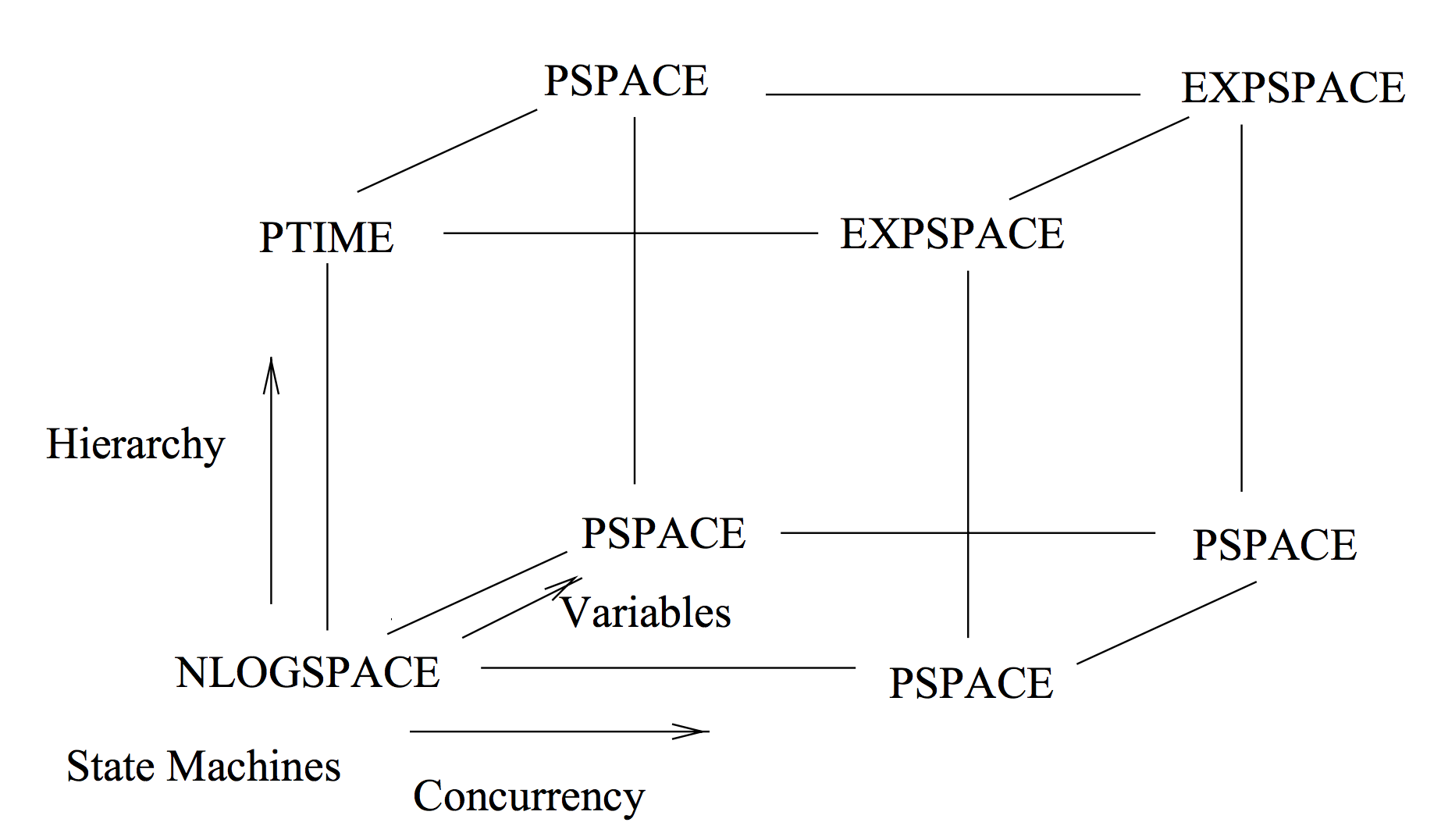In general, abstractions such as variables and concurrency that allow us to create a state space that is the cross product of smaller state spaces, increase succinctness exponentially, but increase verification complexity by the same amount. This is no surprising given the last result.

There actually is one abstraction, called hierarchy, that increases succinctness exponentially, but only increases complexity polynomially in some specific cases. It is used heavily in embedded and RT software, much due to this property, but isn’t common in “plain” programming languages (I believe that subroutines without arguments constitute hierarchy). The simple abstraction of a function, i.e., a subroutine with arguments, is a combination of a variables abstraction with a hierarchy abstraction.

Just as a quick example of why verifying even FSMs is hard, consider a language with nothing but boolean variables and function calls. There are no higher-order functions, no recursion and no iteration provided. Such a language is clearly an FSM language. For example, The number of states in this program is $\leq 2^6$:

A() = B(TRUE) ∧ B(FALSE)
B(a) = C(a, TRUE) ∨ C(a, FALSE)
C(a, b) = D(a, b, TRUE) ∧ D(a, b, FALSE)
D(a, b, c) = E(a, b, c, TRUE) ∨ E(a, b, c, FALSE)
E(a, b, c, d) = F(a, b, c, d, TRUE) ∧ F(a, b, c, d, FALSE)
F(a, b, c, d, e) = X(a, b, c, d, e, TRUE) ∨ X(a, b, c, d, e, FALSE)
X(a, b, c, d, e, f) = ((a ∧ b) ∨ c) ∧ ¬d ∧ (e ∨ f)


Yet, verifying programs in this language is PSPACE-hard, because even the trivial program above directly encodes a TQBF – true quantified boolean formula – problem, which is PSPACE complete (in this case, the formula $\forall a \exists b \forall c \exists d \forall e \exists f . ((a \land b) \lor c) \land \lnot d \land (e \lor f)$).

However, we must point out that the symbolic model-checking result is sensitive to the right-hand side of the model-checking problem: linguistic abstractions do not help verify arbitrary properties, but they may (and do) help create sound state-space reductions that may be used to efficiently verify some particular properties; we’ll briefly discuss those in the next chapter when we mention abstract interpretation. For example, in the simple “TQBF” program above, we can use linguistic abstractions and abstract interpretation to cheaply check whether the value of the variable a may affect the program’s result.

In light of all the results we’ve seen, we can state this informal theorem:

There does not exist a generally useful programming language where every program is feasibly verifiable

So now we know why writing correct programs is hard: because it has to be. But while we cannot verify all programs all the time — regardless of how they’re written — there’s nothing stopping us from verifying some programs some of the time for some properties.

## CHAPTER III: VERIFICATION

Now we know why writing correct programs is hard: because it has to be. But while we cannot verify all programs all the time – regardless of how they’re written – there’s nothing stopping us from verifying some programs some of the time for some properties. As we’ll see, none of the software verification methods manage to overcome the limitations we have explored, and it is precisely those limitations that affect the design and tradeoffs of every verification approach. Of course, software verification is a vast subject, and my goal here is just to give a quick description of the approaches and their relationship to the complexity results we’ve covered.

Turing understood full well the difficulty in writing correct software. This is what he said in his 1947 talk:

[T]he possibilities as to what one may do are immense. One of our difficulties will be the maintenance of an appropriate discipline, so that we do not lose track of what we are doing.

The machine interprets whatever it is told in a quite definite manner without any sense of humour or sense of proportion. Unless in communicating with it one says exactly what one means, trouble is bound to result.

Turing’s solution? Types!

We shall need a number of efficient librarian types to keep us in order.

[this was a joke that didn’t land…]

I mostly mention types because that’s a popular subject among developers who are interested in programming languages, and those turn out to be disproportionately represented in online developer communities who are likely to read this, but also because types can be said to be a form of formal verification and they fit in nicely at the end of the discussion of verification methods.

As a motivation, I’d like to ask the following question. Here’s Rice’s theorem again:

For any non-trivial property of partial functions, no algorithm can decide whether or not a program (TM) M computes a partial function with that property.

Yet, not only is this possible but it takes very little effort to ensure that, say, a C, or Java, or Haskell program returns an int. How is that not a contradiction with the theorem?

Some seem to assume that there is no violation because the property is “correct by construction”. This is false. Rice’s theorem says nothing about construction, and for good reason. Suppose we could easily prove properties on the results of programs by using types, because of “correctness by construction”. We can then have the following efficient algorithm for verifying programs: I write the program in Python, you translate it to, say, Haskell, and voilà! Problem solved! This is impossible, because complexity applies to people as well as to computers.

The real answer must directly address Rice’s theorem: either the property is trivial, or what’s computing the partial function is not a TM.

In the case of an int return type, what it really means is that either the program never terminates or returns an integer. This is a trivial property, as every program either never terminates or returns something that can be interpreted as an integer. Not even a single bit of information is known about the result.

Now, some of you would immediately recognize that this is not always the case. In Haskell, for example, you can relatively easily show that a program’s result is a string of numeric digits. This specifies lots of bits, restricting every byte of output to 10 possible outcomes out of 256. So how is this not a violation of Rice’s theorem? Well, because that property is not generated by a Turing machine, but by a finite state machine, as I’ll now show. But first, we must take a detour to explore some other verification methods.

## Model Checkers

As we’ve seen, for finite state machines that are small enough, there are practical automatic model-checking algorithms. In general, the name model-checker is applied to algorithms that aren’t too reliant on specific syntactic representations of programs.

Modern model checkers use very sophisticated algorithms to explore increasingly large state spaces24, and in some cases infinite ones. Their main advantage is that they are easy to use and extremely general: they actually automatically solve the model-checking problem. Normally, the properties they check are specified in a flavor of temporal logic, which is rich enough to express any program property. Their main downside is that because they solve the model checking problem, they are subject to what’s known as the “state-explosion problem”, which is that even a small program can produce a huge and very irregular state space.

This is an example of the state space of an alternating-bit protocol. The program is about 30 lines long: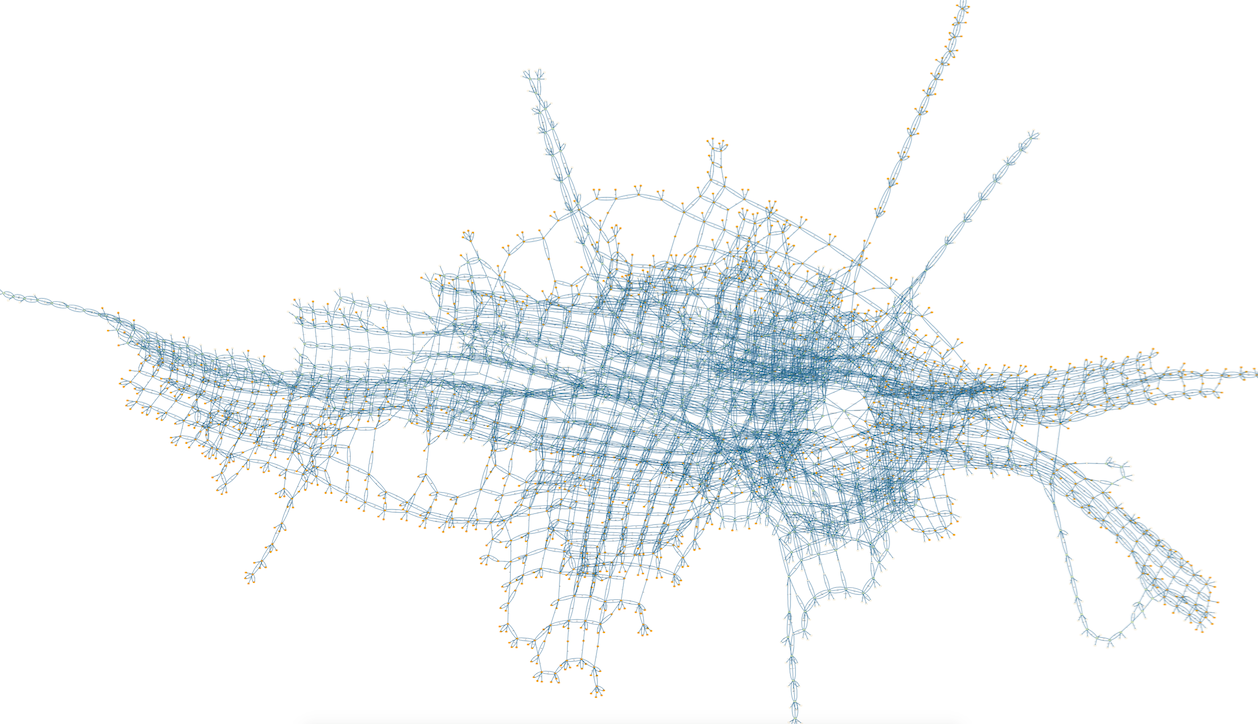And this is the state space of the CAN bus protocol, used in cars: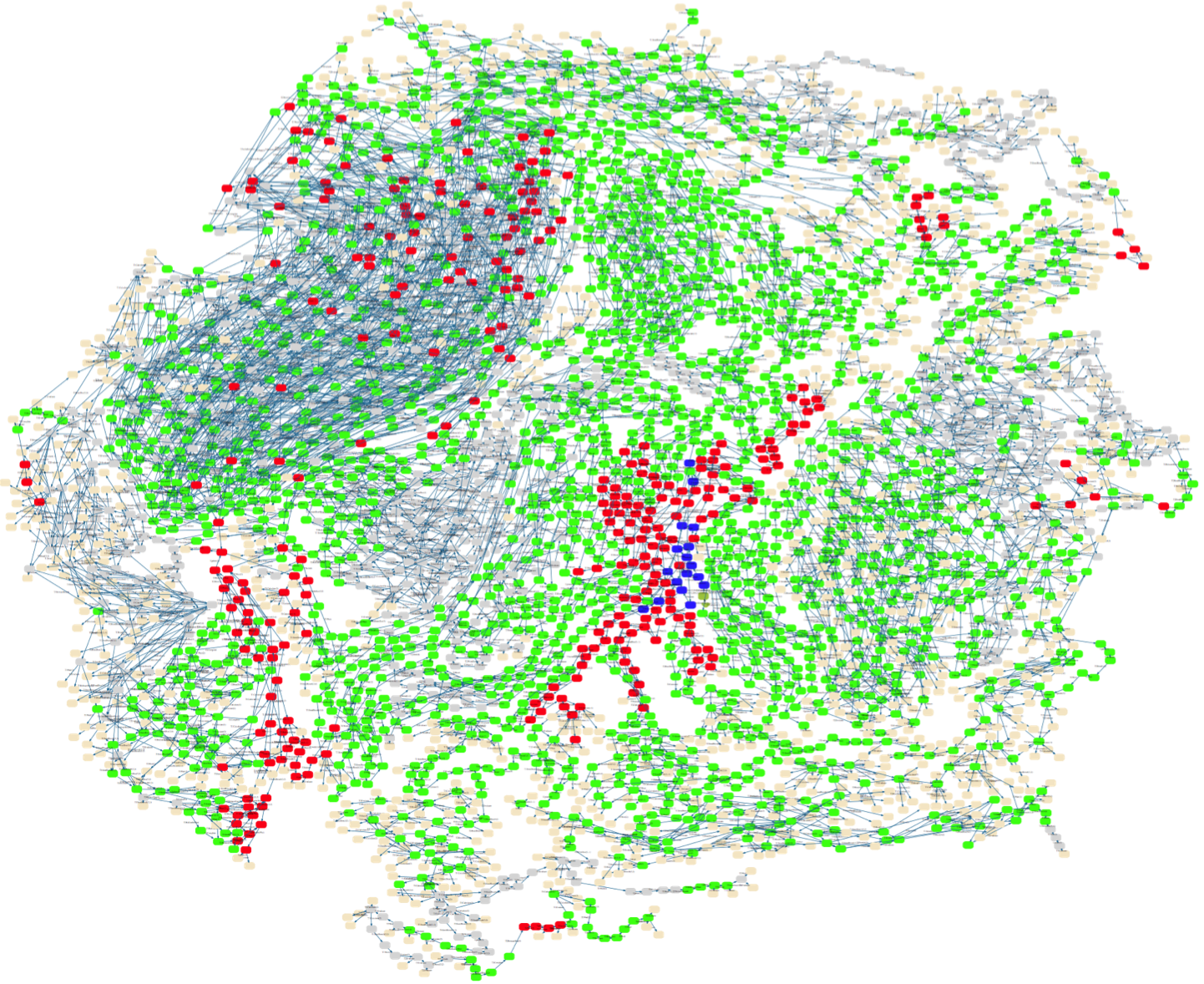Fortunately, most programs requiring the most stringent verification – at least historically – have been rather small (hardware or embedded real-time), and it is therefore no surprise that model-checking is by far the most widely use formal verification method in industry.

It is important to understand that the state-explosion problem affects model checkers not because of the algorithms they use, but because they strive to precisely solve the model-checking problem in the general case – namely all programs, all properties – and so they bear the full brunt of the results we’ve seen ($O(|S|)$).

By the way, the state space of the towers of Hanoi program is a Sierpinski triangle!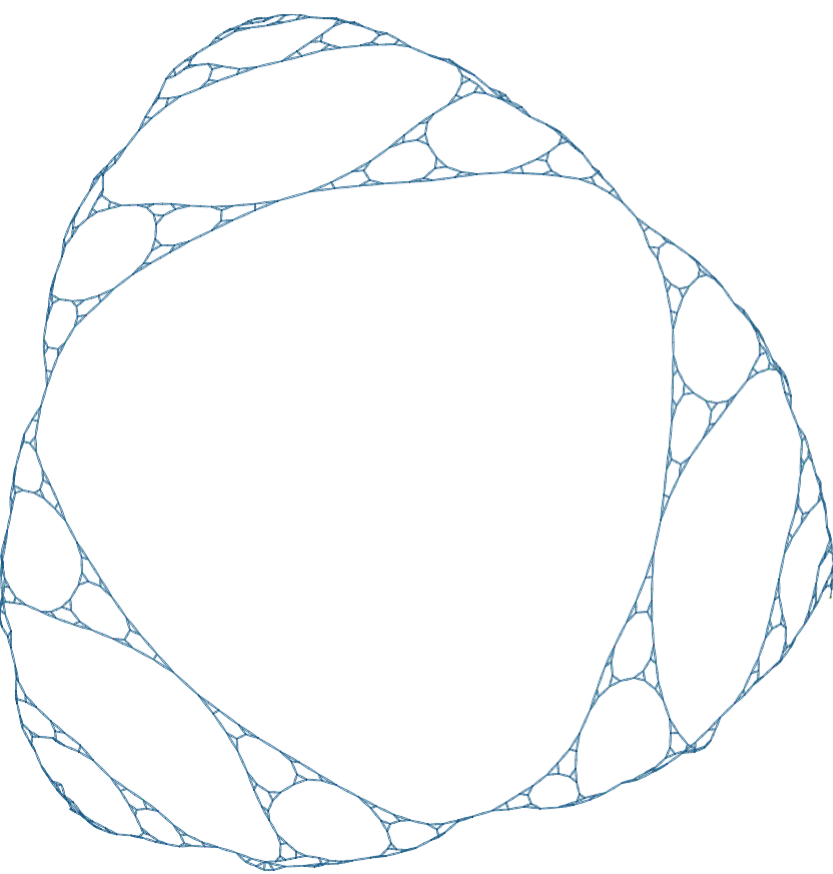More examples can be found on the ProB website.

## Deductive Proofs

Deductive proof (namely, use $M \vdash \varphi$ to show $M \vDash \varphi$) is another general approach. Like model checkers, it is precise, and therefore it is computationally no easier than the model checking problem (in fact, the two can be made equivalent in many cases).

But even though it, too, is subject to the same worst-case complexity bounds it can be more scalable than model-checkers, because it can be tailored to very specific problems, and programs verified with deductive methods are usually written with deductive proofs in mind. Those who use deductive proofs don’t write programs and then prove them. They write and prove hand-in-hand, often severely limiting their program, so that proofs will be possible.

There are interesting advancements in automated proof tools (in particular, SMT solvers), that can assist with many, usually simple theorems, but in general, currently humans do the bulk of the work.

Empirical evidence suggests that this approach – while can be made to work – is very costly, but not more than current high-assurance software development methodologies, used, say, in the defense industry. But now we know why they’re so hard: Someone must pay the complexity price, and if it’s not the computer, it’s us. In the industry, manual deductive proofs are usually used to tie together results from automated proofs and model checkers.

Even if we put aside the difficulty in finding a proof, a feasible proof – i.e. one of polynomial length in the size of the program – may not even exist. Its is a well-known result (by Cook and Reckhow25) that even in the simple propositional calculus – simplest of all logics, just boolean variables and boolean connectives, and, or, not – a short proof exists for all tautologies iff $NP = coNP$, which is assumed to not be the case.

## Abstract Interpretation (Static Analysis)

An elegant and mathematically deep solution to verification is called abstract interpretation, and was introduced by Patrick and Radhia Cousot in the 70s26. Usually tools that employ this technique are called static analysis tools. This technique is fully automatic, but it avoids the intractable complexity bounds by being imprecise (in other words, it may answer a question with “I don’t know”).

The very rough idea is to consider a program that is a sound, but less precise abstraction of our input program, usually as a FSM with a small number of states, which can then be model-checked. The complexity is the $O(|S|)$ of the abstraction. For example, consider the following program:

p0: while (true)
p1:    if isEven(x)
p2:        then x := x div 2
p3:        else x := x + 1


This program has an infinite state space (assuming x is some unbounded integer), but if we only care about the parity of x, we can apply the following transformation to x’s values: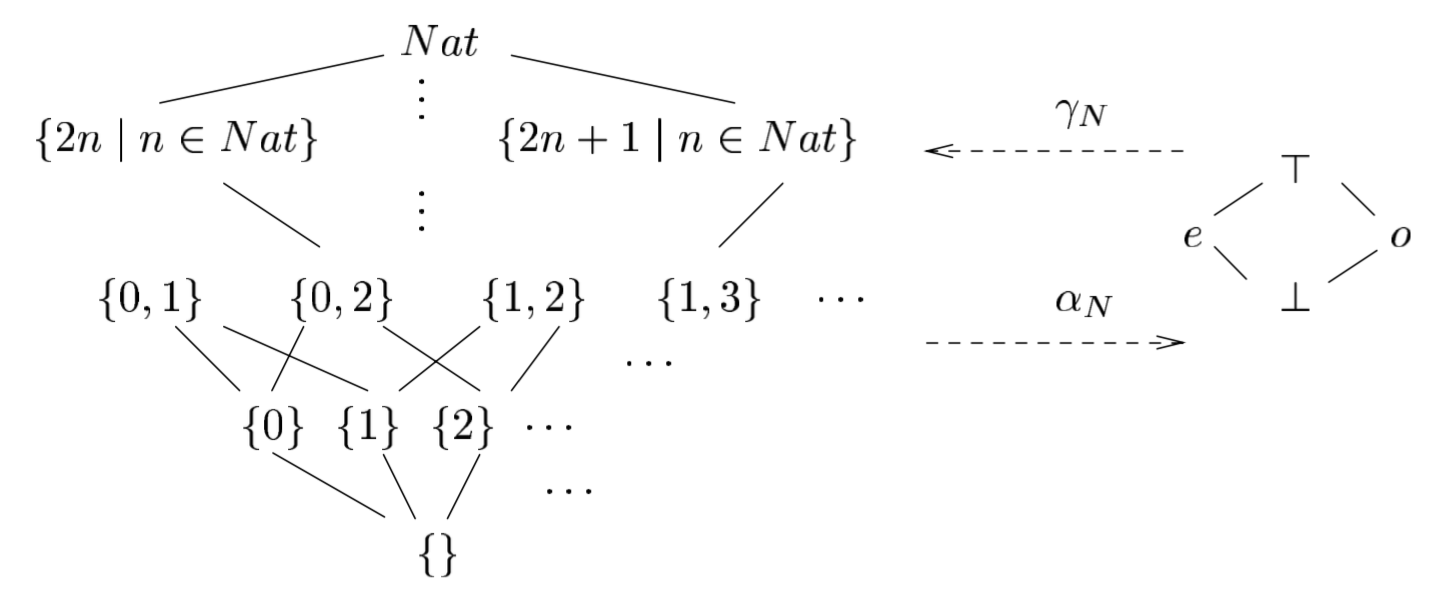and we can then create the following 4-state abstract program, and still know what it is in labels p2, p3: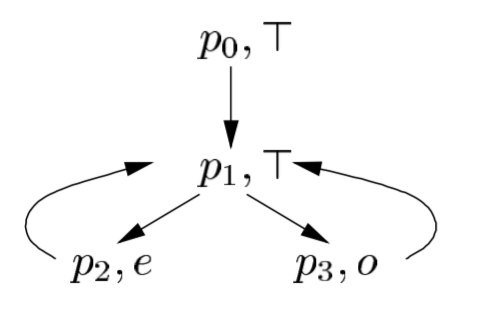The complexity cost of this method would still be – as always – $O(|S|)$, an order of the number of states, but in the abstract program, which is very small (plus the cost of the transformation). The downside, is, of course, that the abstraction may not always capture the properties we’re interested in, and even if it does — not in all circumstances. In the example, we do not know the parity of X in labels p0 and p1.

## Types

One of the several ways of looking at types is as an abstract interpretation27, where a set of values is abstracted as a concrete type. In the abstract program, variables take values which are types. How many states does the abstraction have? About many as there are concrete types in the particular chain of function application; every state is which function we’re applying plus the “current” value, which is a type, so maybe at most the number of concrete types times the number of functions: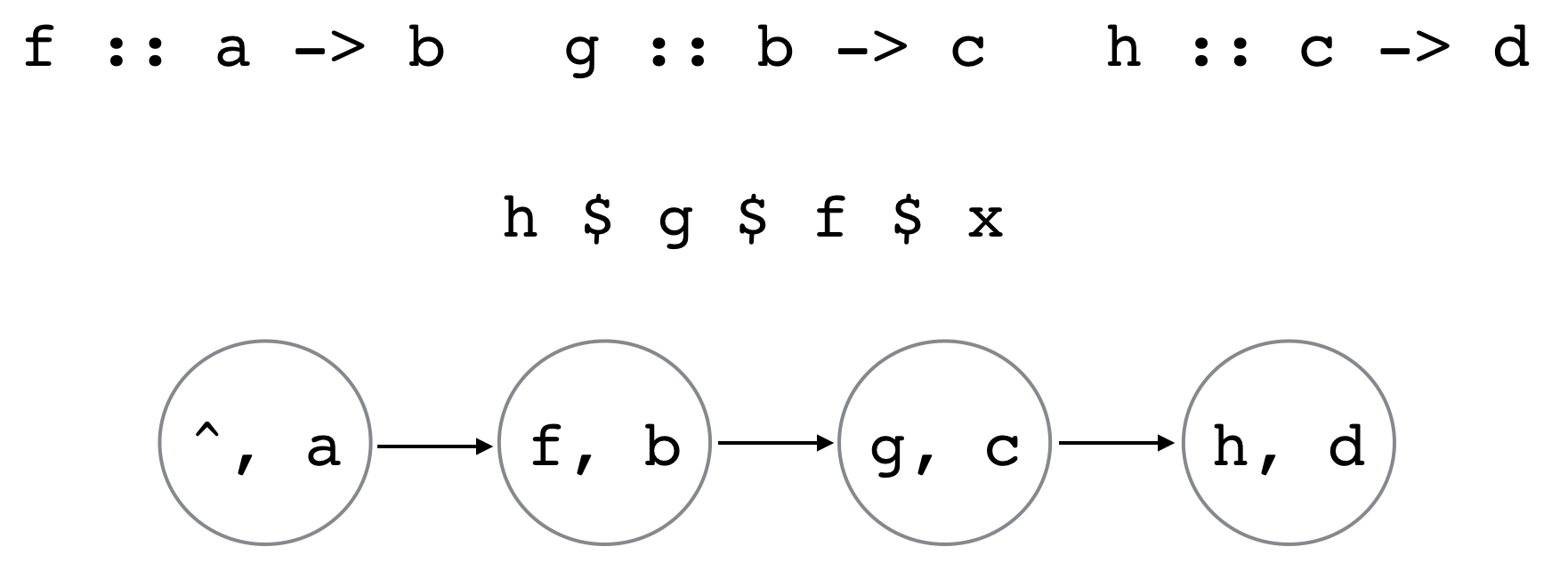The number of concrete types can be greater than the number of types directly spelled out, as programs can have generic types in polymorphic functions, which, in the abstract program, become functions taking a concrete type instance and returning another: just as a concrete function may take the value 3 and return the value 5, an abstract function may take the abstract value Integer and return the value [Integer] (list of integer). I’ll note that the subject of the abstraction created by types is only discussed here in very broad strokes.

So, using nothing but our result of bounded halting, namely $O(|S|)$ and an understanding of abstract interpretation, we can immediately obtain bounds for type-inference algorithms (the algorithm would be $\Omega(\text{# concrete types})$) because type inference requires solving a constraint problem which may be harder).

In a programming language without dependent types (and if you don’t know what they are then it’s not too important), that number is relatively small and is a finite-state machine. The types express properties of FSMs, and so the properties we can prove are not the result of a Turing-complete program, but of a FSM, hence there is no contradiction with Rice’s theorem.

If you need further convincing that non-dependent type systems only encode FSM properties, if you are a Haskell developer you can do the following exercise to convince yourself: try to replace every function body (including library functions, of course) with one that is clearly an FSM by using recursion of constant depth or unbounded depth (depth 0 or 1 should suffice to obtain a type inhabitant in most cases). You’ll find that you can always succeed in replacing a Haskell program with a FSM program that – while completely different from your original program and likely completely wrong – would type-check without requiring any change to any signatures.

CORRECTION: Readers @samth and @kamatsu8 pointed out to me that the above is incorrect when polymorphic recursion is used (which Haskell supports though it does not infer). They provided an example which can only be replaced with a pushdown automaton with a small number of control-states (for which model-checking is also feasible) rather than a FSM.

On the other hand, in languages with dependent types, the number of concrete types can be infinite, and therefore type-inference, which is still $\Omega(|S|)$, is of infinite complexity, i.e., undecidable. It’s really as simple as that.

Those of you who are familiar with the correspondence between types and deductive proofs – called propositions as types or the Curry-Howard correspondence28 – can now understand why deductive proofs can also be viewed as a special case of abstract interpretation, but we won’t explore this notion further here.

To conclude this section, below is a nice slide from a talk by Xavier Leroy29 (author of the formally verified and certified C compiler, CompCert) charting the properties of different formal verification methods by the effort required, the guarantees they provide and the program size they are most suitable for: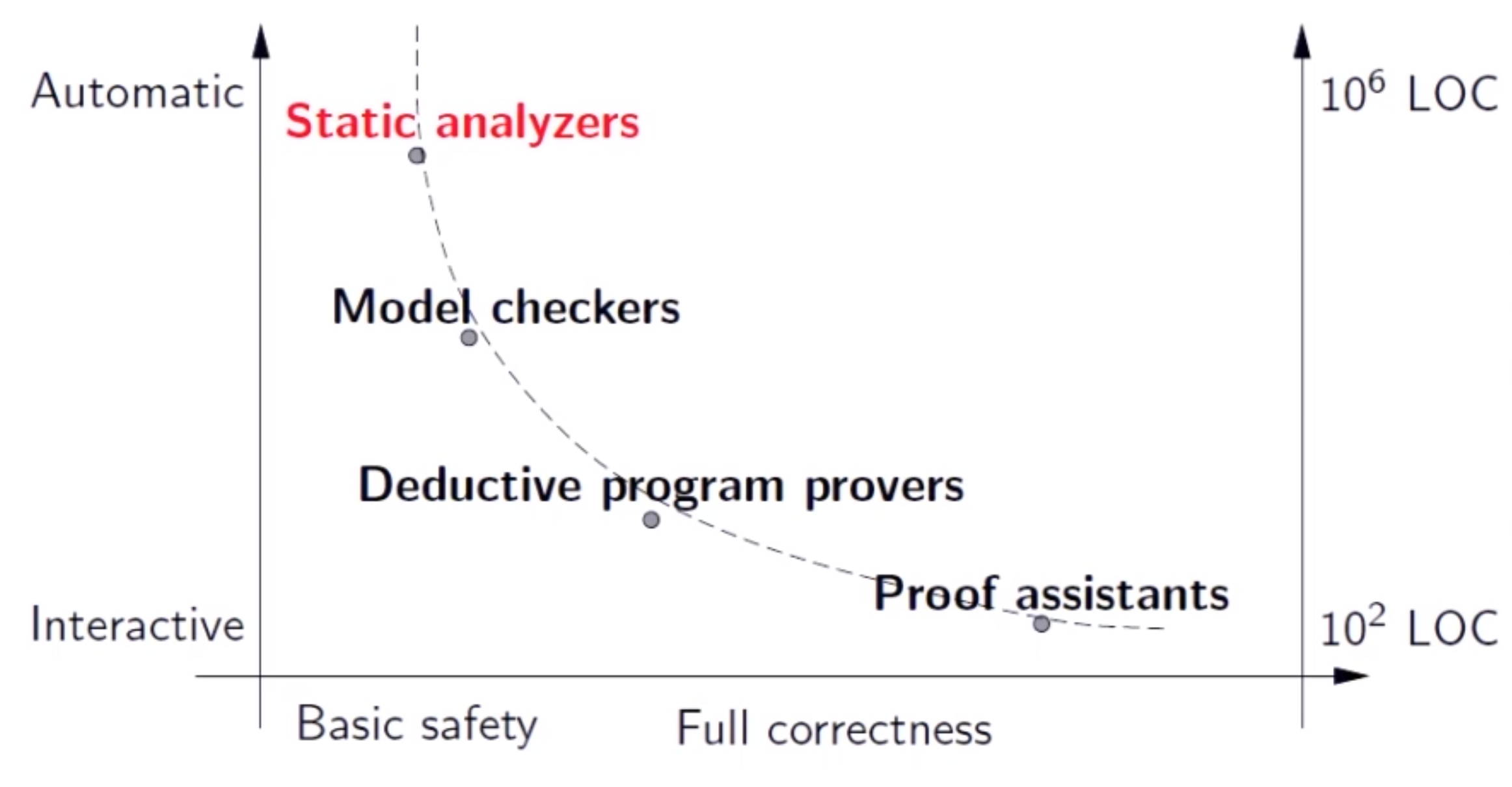## CONCLUSION

In a follow up to his famous article, No Silver Bullet30:, where he argued that we won’t ever see another order-of-magnitude increase in programmer productivity31, Fred Brooks writes:

Is Einstein’s statement that nothing can travel faster than the speed of light “bleak” or “gloomy?” How about Gödel’s result that some things cannot be computed? … [T]he very nature of software makes it unlikely that there will ever be any silver bullets32.

He then quotes Wladyslaw M. Turski, and his take on No Siver Bullet:

Of all misguided scientific endeavours, none are more pathetic than the search for the philosophers’ stone, a substance supposed to change base metals into gold. The supreme object of alchemy, ardently pursued by generations of researchers generously funded by secular and spiritual rulers, is an undiluted extract of wishful thinking, of the common assumption that things are as we would like them to be. It is a very human belief. It takes a lot of effort to accept the existence of insoluble problems. The wish to see a way out, against all odds, even when it is proven that it does not exist, is very, very strong. And most of us have a lot of sympathy for these courageous souls who try to achieve the impossible. And so it continues. Dissertations on squaring a circle are being written. Lotions to restore lost hair are concocted and sell well. Methods to improve software productivity are hatched and sell very well. All too often we are inclined to follow our own optimism (or exploit the optimistic hopes of our sponsors). All too often we are willing to disregard the voice of reason and heed the siren calls of panacea pushers.

I believe that everything we’ve covered in the first two sections is a mathematical proof that Brooks and Turski are right. But what are we to do?

## A Call for Empiricism

We’ve seen that computer science studies objects —- computer programs —- that are composed of very basic components, be they beta reductions in lambda calculus or Turing machine instructions, that are easily subject to full mathematical reasoning on their own, but their interaction is essentially intractable. I think this is similar to physics, where, for example, the gravity equation is very simple, and the equation composes beautifully for multiple instances, and yet even the interaction of three bodies is already chaotic and has no closed form.

I think we should take a page out of physics’s book, and embrace empiricism. Let’s consider the verification problem again: $M \vDash \varphi$

We must restrict either the left hand side or the right hand side. I hope it’s clear by now that it’s unlikely we’ll find a general useful category of programs for which verification is affordable, as verifying even the most simple computational model, the FSM, is in general also infeasible. But we can make the following obvious observation: all programs we care about are created by people. If you like, we can consider humanity to be one large TM that generates all machines $M$. It is likely that patterns could be found in the machines humanity generates that would allow some affordable verification. But because analyzing humanity as a program is truly infeasible, the only viable method is empirical study of the programs humans write. We can begin with a taxonomy of program types, size, cost, etc.

This observation also makes some useful restrictions of the right side possible. So far even the simplest properties we considered (like halting) were global ones. But in practice we know —- empirically! —- that some local properties are also very useful to prove. A great example of that is memory safety, which is known to be a source of common and costly bugs. We may be able to find other useful local properties, but this, too, would require empirical study. A great place to start would be a taxonomy of bugs by cause, prevalence, association with program types, and, most importantly – cost.

Such empirical studies would require the cooperation of academia and industry. With that empirical knowledge in hand, we’ll be able to tackle the interesting special cases using ad-hoc mathematical techniques, just as physics does: simplifying assumptions, heuristics, or approximations.

One such beautiful example of empirical research is a recent study at the university of Toronto, that discovered that a vast majority of catastrophic failures in large distributed system is due to programmers not putting enough thought into error-handling code. This is clearly a psychological effect.

Almost all (92%) of the catastrophic system failures are the result of incorrect handling of non-fatal errors explicitly signaled in software in 58% of the catastrophic failures, the underlying faults could easily have been detected through simple testing of error handling code33.

They studied catastrophic failures in distributed systems, and discovered that a large number of errors is a result of people not putting any thought into exception-handling code. This is clearly a psychological phenomenon of humans writing software. The researchers then created a simple linter that identifies this dangerous pattern. The simple tool would have prevented 33% of all catastrophic failures in Cassandra, HBase, HDFS, and MapReduce.

Computer science isn’t physics, but it isn’t math, either. Juris Hartmanis writes:

Computer science differs from the known sciences so deeply that it has to be viewed as a new species among the sciences34.

## Complexity is Essential!

I’ll conclude with something Fred Brooks wrote: “Complexity is the business we are in, and complexity is what limits us.”

Yet, just as Turski recognized, some people seem to be philosophically offended by this notion, and try to fight the proven limitations. They are under the impression that the world — and I’m not just talking about computing now — is essentially simple, and it the stupidity of people and institution that needlessly complicate it (or, in the case of software, “stupid” programming languages). If we apply careful mathematical reasoning, we could find solutions to anything.

If I were to sum up computational complexity theory in a sentence it would be, “there are no easy solutions to hard problems”. If we were to summarize the results presented here, that would be, “… and if your problem is to predict, author or direct a computational process, then it is hard.”

Computer science is the very discipline that proved that essential complexity can arise even in the smallest of systems. Yet sometimes it is computer scientists and software developers who attempt to challenge the very foundation of their own discipline. Complexity is essential. It cannot be tamed, and there is no one big answer. The best we can do — in society as in computing — is apply lots of ad hoc techniques, and, of course, to try to learn about the particular nature of problems as much as possible.

## Selected Bibliography

The entries are ordered by their order of mention in the article/talk. The full list of references appears in the footnotes below.

At the conference, I was happy to see some researchers stressing the necessity of empirical research (or at least consideration for empirical observations) of industry software development and ad hoc solutions. Some of the talks containing this theme were:

Also, make sure to watch this cool talk/demo of Truffle/Graal.

1. John W. Dawson, Jr., Logical Dilemmas, The Life and Work of Kurt Gödel, A K Peters,1997 p. 71

2. Dawson, p.69

3. Saul Kripke, The Origins and Nature of Computation, Lecture at Tel Aviv University, June 13, 2006, 1:45:50-1:47:10

4. Alonzo Church, An Unsolvable Problem of Elementary Number Theory, 1936

5. A. M. Turing, On Computable Numbers, with an application to the Entschidungsproblem, 1936

6. Scott Aaronson, Rosser’s Theorem via Turing machines, July 2011

7. To see that reachability is no harder than halting, we can take an arbitrary reachability problem, replace all the existing halting states with infinite loops, and mark the state we want to test reachability for as halting. Solving the halting problem would be identical to solving the reachability problem, as the transformed program would halt if-and-only-if the tested state was reachable in the original program.

8. Tibor Rado, On Non-Computable Functions, 1961

9. Adam Yedidia and Scott Aaronson A Relatively Small Turing Machine Whose Behavior Is Independent of Set Theory, 2016

10. Although the subject of infinity in mathematics has always been controversial, with some logicians arguing against it, I would say that the reason for the opposition is precisely because of the same physical limitations that we associate with computation.

11. A. M. Turing, A lecture to the London Mathematical Society, February 20, 1947

12. Some readers may object. After all, programs are abstract mathematical concepts. But just as physics, as an academic discipline, is not concerned with the study of all properties of all physical objects (or chemistry and biology wouldn’t exist as separate disciplines), the academic discipline of mathematics need not be concerned with the study of all mathematical objects (of course, math as a tool is used extensively in computer science, just as it is used in physics, chemistry and biology).

13. Jack Edmonds, Paths, Trees, and Flowers, 1965

14. A panel on BBC radio, recorded January 10, 1952, as appears in, B. Jack Copeland (Editor), The Essential Turing: Seminal Writings in Computing, Logic, Philosophy, Artificial Intelligence, and Artificial Life: Plus The Secrets of Enigma, Oxford University Press, 2004, p. 503  2

15. J. Hartmanis and R.E. Stearns, On The Computational Complexity of Algorithms, 1965

16. Juris Hartmanis, Turing Award Lecture: On Computational Complexity and the Nature of Computer Science, 1994

17. Stephen A. Cook and Robert A. Reckhow, Time Bounded Random Access Machines, 1972

18. D. A. Turner, Total Functional Programming, 2004

19. I should note that totality – especially in functional languages – may serve some useful purposes; making verification easier, however, is not one of them.

20. Stephen Bellantoni and Stephen Cook, A New Recursion-Theoretic Characterization of the Polytime Functions, 1992

21. S. Demri, F. Laroussinie and Ph. Schnoebelen, A Parametric Analysis of the State-Explosion Problem in Model Checking, 2005

22. Philippe Schnoebelen, The Complexity of Temporal Logic Model Checking, 2002  2

23. Rajeev Alur, Formal Analysis of Hierarchical State Machines, 2003

24. Edmund M. Clarke, Model checking: my 27-year quest to overcome the state explosion problem, 2007 and Progress on the State Explosion Problem in Model Checking, 2001

25. Stephen A. Cook and Robert A. Reckhow, The Relative Efficiency of Propositional Proof Systems, 1979

26. For an introduction, see Patrick Cousot, Abstract Interpretation Based Formal Methods and Future Challenges, 2001. For a fascinating, readable overview of the general theory of abstraction and refinement, see David A. Schmidt, Binary Relations for Abstraction and Refinement, 1999.

27. Type system experts may wish to see the following two papers: Patrick Cousot, Types as Abstract Interpretations, 1997 and Roberta Gori, Giorgio Levi, An experiment in type inference and verification by abstract interpretation, 2002. These papers are well beyond my understanding.

28. For a thorough overview, see Jean-Yves Girard, Proofs and Types, 1989

29. Xavier Leroy, Desperately seeking software perfection, October 20, 2015

30. F. P. Brooks, No Silver Bullet: Essence and Accidents of Software Engineering, 1986

31. Actually, Brooks wrote, “There is no single development, in either technology or management technique, which by itself promises even one order-of-magnitude improvement within a decade in productivity, in reliability, in simplicity.” Given that three decades have passed without an order-of-magnitude increase in productivity even taking all developments combined, and given Brooks’s later treatment of his informal prediction as a law of nature, I’ve allowed myself to paraphrase for simplification.

32. F. P. Brooks, No Silver Bullet, Refired, The Mythical Man-Month, Essays on Software Engineering, Anniversary Edition, Addison-Wesley 1995, p.213

33. Juris Hartmanis, Some Observations About the Nature of Computer Science, 1993